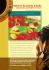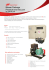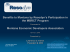# Multi-frame factorisation for long

## Transcription

Multi-frame factorisation for long
```MULTI-FRAME FACTORISATION FOR LONG-SPAN ACOUSTIC MODELLING
Liang Lu and Steve Renals
Centre for Speech Technology Research, University of Edinburgh, Edinburgh, UK
{liang.lu, s.renals}@ed.ac.uk
ABSTRACT
Acoustic models based on Gaussian mixture models (GMMs)
typically use short span acoustic feature inputs. This does not
capture long-term temporal information from speech owing to
the conditional independence assumption of hidden Markov
models. In this paper, we present an implicit approach that
approximates the joint distribution of long span features by
product of factorized models, in contrast to deep neural networks (DNNs) that model feature correlations directly. The
approach is applicable to a broad range of acoustic models.
We present experiments using GMM and probabilistic linear
discriminant analysis (PLDA) based models on Switchboard,
observing consistent word error rate reductions.
Index Terms— Acoustic modelling, long span features,
multi-frame factorisation
1. INTRODUCTION
Hidden Markov model (HMM) based speech recognition 
typically uses acoustic feature vectors that are extracted from
a short temporal window of context at a fixed frame rate.
These acoustic feature vectors are assumed conditionally
independent given the HMM state sequence, which allows
efficient acoustic model training and decoding. However, this
has been viewed as one of major weaknesses of HMM-based
acoustic models , because there is significant temporal
dependence between neighbouring acoustic frames – a dependency that cannot be captured efficiently by conventional
HMM-based acoustic models.
Conventional HMM systems, using Gaussian mixture
model (GMM) output distributions, do incorporate some
acoustic context, for example through the use of dynamic
features  or through the projection of multiple acoustic
frames using linear discriminant analysis . These approaches work well in practice, although they violate the
dependence assumptions of the HMM. Since the additional
dynamic features are arrived at through a linear transform of
the original feature vector sequence, it is possible to interpret
the resultant acoustic models as defining a probability over
the complete observation sequence. This may be considered
Funded by EPSRC Programme Grant EP/I031022/1 (Natural Speech
Technology).
as a different model, referred to as the trajectory HMM, and
the training and decoding algorithms can be derived accordingly .
Segment-based acoustic models [6, 7] aim to model subword units – such as phones or sub-phones – directly. These
approaches model the joint distribution of variable-length observation sequences (with or without the conditional independence assumption) and the segment models are used as the
basic building blocks, rather than HMM states. More recently segmental conditional random fields (CRFs)  have
been proposed. Segmental CRFs differ from the previously
proposed models through the use of multi-scale detectors to
extract segmental-level features, using different CRF feature
functions which are integrated to predict the state sequence.
Neural network (NN) acoustic models have achieved
considerable success using concatenated acoustic vectors
from wider windows of context to take advantage of the
long temporal acoustic information for classification. These
approaches have resulted in improvements in accuracy in
both hybrid NN/HMM systems [9, 10, 11, 12, 13] and in
GMM-based systems using tandem or bottleneck features obtained from NNs trained for context-independent or contextdependent phone classification [14, 15, 16]. In the case
of tandem or bottleneck features, the HMM independence
assumptions are again violated (and accuracy is again improved).
In this paper, we investigate generative acoustic models
which directly model multiframe context. Modelling the joint
distribution of concatenated features is normally prohibitive
for generative models owing to the high dimensionality, and
applying explicit dependency between multiple frames – such
as in the autoregressive HMM  and linear dynamic systems
 – makes efficient inference difficult. We consider a simple approach which factorizes the joint distribution of multiple frames by a product of the probabilities of the individual
frames. This can be viewed as a type of segment model that
uses a fixed length segmentation, thus avoiding the need to
estimate latent segmentation variables. This approach is very
general and is applicable to a wide range of acoustic models. In this paper, probabilistic linear discriminant analysis
(PLDA)  (cf. Section 4) is used as an example. Our experiments on Switchboard demonstrate that the approach results
in reductions in word error rate (WER).
2. HIDDEN MARKOV MODEL AND
LONG-SPAN ACOUSTIC MODELLING
and if we do not consider the effect of feature overlapping
when computing the state transition probability, this model
may be represented as
2.1. Hidden Markov Model
Given a sequence of acoustic observations of length T , O =
ˆ is obtained by
(o1 , · · · , oT ), the optimal word sequence W
the maximum a posteriori (MAP) decoding rule as
ˆ = arg max p(O|M, W)P (W)
W
W
(1)
where M denotes the acoustic model parameters, and P (W)
is the prior probability obtained from a language model. Using HMMs, the acoustic likelihood score is computed as
X
p(O|q, M)P (q)
(2)
p(O|M, W) =
q∈ΦW
where q = (q0 , q1 , · · · , qT , qT +1 ) denotes an HMM state sequence corresponding to the observations, and ΦW denotes
the set of all the possible HMM state sequences of W. q0
and qT +1 are non-emitting entry and exit states that are not
considered in the following for simplicity. Equation (2) can
be solved efficiently if we apply the first-order Markov and
conditional independence assumptions:
P (q) ≈
T
Y
P (qt |qt−1 ),
p(O|q, M) ≈
t=1
T
Y
p(ot |q, M)
t=1
p(O|M, W) ≈
T
X Y
p(yt |qt , M)P (qt |qt−1 )
(7)
q∈ΦW t=1
where 2k + 1 is the context size. Though it is not very precise theoretically, this approach works well for DNN-based
acoustic models [11, 12]. In practice, the short span features
{ot+n , n ∈ [−k, k]} may overlap with each other in the time
domain, and if ot+n is beyond the utterance length, the empty
elements can be padded by the first or last frames.
Using long span features such as yt is impractical for
GMM-based acoustic models due to the expansion of model
size and diagonalisation of covariance. DNNs, however, do
not have this difficulty, and the scaled likelihood can be computed efficiently as
p(yt |qt , M) ∝ P (qt |yt , M)/P (qt )
(8)
Previously, we studied the use of PLDA to estimate the joint
probability distribution p(yt |qt , M) directly which is able
capture feature correlations and is more scalable to higher
dimensional features . However, this approach does not
work well for very high dimensional features. In this paper,
we investigate an implicit approach which approximates this
joint distribution by product of factorized models.
(3)
3. MULTI-FRAME FACTORISATION
Given that, we can rewrite equation (2) as
q∈ΦW t=1
The idea of factorizing the distribution of p(yt |qt , M) is not
new. For instance, the semi-parametric trajectory model ,
rewrites the joint distribution as
which is behind the HMM-based acoustic models.
p(yt |qt , M) = p(τ t |ot , qt , Mτ )p(ot |qt , Mo )
T
X Y
p(O|M, W) ≈
p(ot |qt , M)P (qt |qt−1 )
(4)
2.2. Long-span acoustic modelling
To capture long term dependence, one way is to address the
conditional independence assumption of HMMs directly. For
instance, some Bayesian models introduce an explicit dependency between observations in equation (3), e.g.
p(O|q, M) ≈
T
Y
p(ot |ot−1 , q, M)p(o1 |q, M)
(5)
t=2
Typical examples include autoregressive HMM  and
switching linear dynamic systems . However, these models are usually expensive to train, and do not work well in
practice.
Another approach is to use long span features directly by
concatenating the observations of certain context size without
formulating the internal dependence explicitly. If we define
where τ t := (ot−k , . . . , ot−1 , ot+1 , . . . , ot+k ), and we have
used Mτ , and Mo to show that the two distributions may be
modelled by different sets of model parameters. In , the
model is simplified by dropping the dependency on ot as
p(τ t |ot , qt , Mτ ) ≈ p(τ t |qt , Mτ )
(6)
(10)
In this case the factorisation is performed on each Gaussian
component for a GMM-based acoustic model; here we do not
introduce a Gaussian component index, in order to clarify the
presentation of the basic idea.
In this paper, we present a multi-frame model which factorizes the joint probability distribution into a product of probabilities of each individual frames. This can be written as
k
Y
p(yt |qt , M) ≈
pγn (ot+n |qt , Mn )
(11)
n=−k
s.t.
yt := (ot−k , . . . , ot , . . . , ot+k )
(9)
X
n
γn = 1
(12)
where Mn denotes the parameters that model the distribution of the individual frames ot+n , and γn is the scaling factor which normalises the distribution and compensates for
any factorisation error. Compared to standard HMMs (equation (7)), this approach predicts the states by averaging scores
from a wider context. Note that the factorisation can be performed at the state level or the Gaussian component level .
This approach does not rely on a particular type of acoustic model, and a similar approach may be applied to DNNs
, where the state posterior probability is obtained as
p(qt |yt , M) ≈
k
Y
pγn (qt |ot+n , Mn )
(13)
n=−k
1
, and here ot−n itself is a long span feature
where γn = 2k+1
vector, as normally used in DNNs. The authors report considerably improved accuracy using this approach . In this
paper, we show that the multi-frame factorisation approach
is also applicable to generative models. Note that in (11)
some model parameters for each short span features Mn
may be shared: for instance, the hidden layers of DNNs may
be shared across all the factorized models as in  , while
for structured generative models such as the subspace GMM
(SGMM)  and PLDA , the state-independent model
parameters can be tied across all the factorized models, which
is similar to multilingual training.
4. PLDA-BASED ACOUSTIC MODEL
As discussed before, multi-frame factorisation is applicable to
general types of acoustic models including DNN hybrid models, e.g. . In this paper, we apply the approach to a generative model based on PLDA. Our motivation is that the PLDA
acoustic model is more flexible with respect to higher dimensional features compared to GMMs, allowing us to compare
the results of modelling the joint distribution p(yt |qt , M) directly to multi-frame factorisation.
The basic idea behind PLDA acoustic models is that the
distribution over acoustic feature vectors yt ∈ Rd from the
j-th HMM state at time t (i.e. qt = j) is expressed as :
yt |j = Uxjt + Gzj + b + jt ,
jt ∼ N (0, Λ) .
(14)
zj ∈ Rq is the state variable shared by the whole set of acoustic frames generated by the j-th state and xjt ∈ Rp is the
frame variable which explains the per-frame variability. Usually, the dimensionality of these two latent variables is smaller
than that of the feature vector yt , i.e. p, q ≤ d. U ∈ Rd×p
and G ∈ Rd×q are two low rank matrices which span the subspaces to capture the major variations for xjt and zj respectively. b ∈ Rd denotes the bias and jt ∈ Rd is the residual
noise which is assumed to be Gaussian with zero mean and
diagonal covariance. By marginalising out jt and xjt , we
obtain the following likelihood function:
p(yt |j) = N (yt ; Gzj + b, UUT + Λ)
(15)
To increase the modelling capacity, we presented a tied PLDA
mixture model , which computes the state likelihood as
X
p(yt |j) =
wjkm N yt ; Gm zjk + bm , Um UTm + Λm
mk
where k denotes the sub-state index, and zjk is the sub-state
variable, cjk is the sub-state weight, πjm is the component
weight which is shared for all the sub-state models, and
wjkm = cjk × πjm . This model is closely related to the
SGMM , and more details can be found in .
5. EXPERIMENTS AND DISCUSSION
We performed experiments using the Switchboard corpus
. The Hub-5 Eval 2000 data  is used as the test
set, which contains the Switchboard (SWB) and CallHome
(CHM) evaluation subsets. The experiments were performed
using the Kaldi speech recognition toolkit , and we have
used GMM- and tied PLDA-based acoustic models. In the
following experiments, we used maximum likelihood estimation without speaker adaptation. The pronunciation lexicon
was obtained from the Mississippi State transcriptions 
and a trigram language model was used for decoding.
5.1. Baseline systems
Table 1 shows the WERs of the baseline systems, trained using about 33 hours of Switchboard training data. The number
of tied HMM states is around 2,400 for each of the acoustic models shown in this table. The GMM system has about
30,000 Gaussian components. In the PLDA system, the state
and frame variables are both 40-dimensional, irrespective of
can be found in . Since the PLDA acoustic model is more
flexible with respect to feature vectors dimension, we evaluated the effect of long span features by splicing 13-dimension
MFCC 0 of different context size. Table 1 shows that these
features can improve the accuracy of PLDA systems, however, no further improvement was achieved when the feature
length is longer than MFCC 0(±3), i.e. splicing 3 left/right
frames with the current one. Previously, we used spliced
MFCC 0 ∆ ∆∆ as features but did not obtain better results
. As a comparison, we also show the WERs of GMM systems using the same long span features but with feature space
linear discriminant analysis (LDA) which reduces the feature
dimensionality to 40 and followed by semi-tied covariance
matrix (STC) modelling . Table 1 shows that the PLDA
systems consistently outperform their GMM counterparts.
5.2. Results of using multi-frame factorisation
Based on our previous results, we use MFCC 0(±3) as the
basic feature unit ot in the following experiments to evaluate
the multi-frame factorisation using PLDA. Before presenting
Table 2. Results of using multi-frame factorisation for PLDA
systems using different feature context n ∈ [−k, k].
WER (%)
32.5
32
31.5
31
tï2
tï1
t
Switchboard
t+1
t+2
48.8
WER (%)
48.6
48.4
System
Baseline
MF-State
MF-State
Baseline
MF-State
MF-State
MF-Sequence
MF-Sequence
k
0
1
2
0
1
2
1
2
Decoding
MAP
MAP
MAP
MBR
MBR
MBR
MBR
MBR
CHM
47.9
47.3
48.4
47.2
46.6
47.9
46.2
45.8
SWB
31.0
30.3
30.8
30.3
29.7
30.3
29.6
29.4
Avg
39.5
38.8
39.7
38.9
38.3
39.3
37.9
37.7
48.2
Table 3. Results of using 300 hours of training data.
48
47.8
tï2
tï1
t
CallHome
t+1
t+2
Fig. 1. WERs of systems p(ot+n |qt , M), n ∈ [−2, 2] on the
Switchboard and CallHome evaluation set.
Table 1. WER(%) of GMM and PLDA baseline systems using 33 hours of training data.
System
GMM
GMM
GMM
GMM
GMM
PLDA
PLDA
PLDA
PLDA
Feature
MFCC 0+∆+∆∆
MFCC 0(±2)+LDA
MFCC 0(±3)+LDA
MFCC 0(±4)+LDA
MFCC 0(±5)+LDA
MFCC 0 (±2)
MFCC 0 (±3)
MFCC 0 (±4)
MFCC 0 (±5)
STC
STC
STC
STC
CHM
54.0
54.4
50.6
50.7
50.9
48.6
47.9
47.5
48.7
SWB
36.6
34.4
33.5
33.3
34.1
31.9
31.0
31.2
32.2
Avg
45.4
43.7
42.2
42.1
42.4
40.4
39.5
39.4
40.6
these results, we first evaluate the systems that use individual
feature frames, i.e. the state likelihood score is computed as
p(ot+n |qt , Mn ), n ∈ [−k, k]. These systems were trained in
the usual way except that given the alignment, we used ot+n
instead of ot to accumulate statistics for qt to train model parameters. The aim is to study the effect of mismatch between
the alignments and feature inputs, and the correlations between the consecutive frames. In addition, these systems are
also baselines for the multi-frame factorisation system since
it averages the scores from those systems. These results are
shown in Figure 1. We observe that shifting the feature input
by 1 time step results in a marginal performance degradation,
otherwise the systems obtained much worse results. It will be
interesting to see if this trend holds for DNNs.
We then used multi-frame factorisation to integrate the
factorized models using (11), which is referred to as MF-State
in Table 2 because the factorisation is performed at the state
level. In this work, the scaling factors γn were simply set
1
. However, their values can either be tuned manto be 2k+1
ually or learned by Bayesian optimisation approach .
As a comparison, we also performed system combination
using minimum Bayes risk (MBR) decoding by combining
the word lattices from each sub-system , which is re-
System
Baseline
MF-State
MF-State
Baseline
MF-State
MF-State
MF-Sequence
MF-Sequence
k
0
1
2
0
1
2
1
2
Decoding
MAP
MAP
MAP
MBR
MBR
MBR
MBR
MBR
CHM
40.8
40.2
41.3
40.2
39.7
40.8
39.2
39.1
SWB
25.1
24.6
25.5
24.6
24.3
25.2
24.2
24.1
Avg
33.1
32.5
33.5
32.6
32.2
33.2
31.8
31.7
ferred as MF-Sequence. Since MBR decoding itself may
perform better than MAP, we show both results for baseline
systems for strict comparison. Table 2 shows that although
the sub-systems in Figure 1 are worse than the baseline, both
state and sequence level integration result in lower WER.
The MF-State system does not achieve better results when
k = 2 because the sub-systems trained on ot−2 and ot+2
are very poor, and using equal scaling factors γn is not optimal. However, this system still works much better than
that without factorisation which corresponding to PLDA–
MFCC 0(±5) system in Table 1. These results indicate that
the factorisation approach can work better than directly estimate the joint distribution of long span features. A similar
trend was observed when using 300 hours of training data as
shown by Table 3. In future, we shall investigate using wider
feature context and factorisation approach (13) for DNNs.
6. CONCLUSION
In this paper, we study multi-frame factorisation for long-time
acoustic modelling where the distribution of long-span acoustic features is factorized into a product of short-time models.
Compared to approaches that apply explicit dependency between observation for long temporal modelling, this approach
is more efficient in model training, and is applicable to a
wide range of acoustic models. Experiments on Switchboard
demonstrate that this approach can improve speech recognition accuracy. The factorisation approach presented in this
paper is very simple. In the future, we shall investigate approaches to reduce the factorisation error and to approximate
the joint distribution more precisely.
7. REFERENCES
 L. Rabiner, “A tutorial on hidden Markov models and selected
applications in speech recognition,” Proceedings of the IEEE,
vol. 77, no. 2, pp. 257–286, 1989.
 D. Gillick, L. Gillick, and S. Wegmann, “Don’t multiply
lightly: Quantifying problems with the acoustic model assumptions in speech recognition,” in Proc. ASRU.
IEEE,
2011, pp. 71–76.
 S. Furui, “Speaker-independent isolated word recognition using dynamic features of speech spectrum,” IEEE Trans on
Acoustics, Speech, and Signal Processing, vol. 34, pp. 52–59,
1986.
 G. Saon, M. Padmanabhan, R. Gopinath, and S. Chen, “Maximum likelihood discriminant feature spaces,” in Proc. IEEE
ICASSP, vol. 2, 2000, pp. 1129–1132.
 H. Zen, K. Tokuda, and T. Kitamura, “Reformulating the
HMM as a trajectory model by imposing explicit relationships
between static and dynamic feature vector sequences,” Computer Speech and Language, vol. 21, pp. 153–173, 2007.
 M. Ostendorf, V. V. Digalakis, and O. A. Kimball, “From
HMM’s to segment models: A unified view of stochastic modeling for speech recognition,” Speech and Audio Processing,
IEEE Transactions on, vol. 4, no. 5, pp. 360–378, 1996.
 J. R. Glass, “A probabilistic framework for segment-based
speech recognition,” Computer Speech & Language, vol. 17,
no. 2, pp. 137–152, 2003.
 G. Zweig and P. Nguyen, “A segmental CRF approach to large
vocabulary continuous speech recognition,” in Proc. ASRU.
IEEE, 2009, pp. 152–157.
 H. A. Bourlard and N. Morgan, Connectionist speech recognition: a hybrid approach. Springer, 1994, vol. 247.
 N. Morgan, Q. Zhu, A. Stolcke, K. Sonmez, S. Sivadas, T. Shinozaki, M. Ostendorf, P. Jain, H. Hermansky, D. Ellis, G. Doddington, B. Chen, O. Cetin, H. Bourlard, and M. Athineos,
“Pushing the envelope - aside,” IEEE Signal Processing Magazine, vol. 22, no. 5, pp. 81–88, 2005.
 F. Grezl, M. Karafiat, S. Kontar, and J. Cernocky, “Probabilistic and bottle-neck features for LVCSR of meetings,” in Proc.
IEEE ICASSP, vol. 4, 2007, pp. 757–760.
 J. Droppo and A. Acero, “Noise robust speech recognition with
a switching linear dynamic model,” in Proc. ICASSP, vol. 1.
IEEE, 2004, pp. I–953.
 L. Lu and S. Renals, “Probabilistic linear discriminant analysis
for acoustic modelling,” IEEE Signal Processing Letters, 2014.
 S. Liu and K. C. Sim, “Temporally varying weight regression: A semi-parametric trajectory model for automatic speech
recognition,” IEEE/ACM Transactions on Audio, Speech and
Language Processing (TASLP), vol. 22, no. 1, pp. 151–160,
2014.
 N. Jaitly, V. Vanhoucke, and G. Hinton, “Autoregressive product of multi-frame predictions can improve the accuracy of hybrid models,” in Proc. INTERSPEECH, 2014.
 D. Povey, L. Burget, M. Agarwal, P. Akyazi, F. Kai,
A. Ghoshal, O. Glembek, N. Goel, M. Karafi´at, A. Rastrow,
R. Rose, P. Schwarz, and S. Thomas, “The subspace Gaussian
mixture model—A structured model for speech recognition,”
Computer Speech & Language, vol. 25, no. 2, pp. 404–439,
2011.
 L. Lu and S. Renals, “Tied probabilistic linear discriminant
analysis for speech recognition,” arXiv:1411.0895 [cs.CL],
2014.
 J. J. Godfrey, E. C. Holliman, and J. McDaniel, “SWITCHBOARD: Telephone speech corpus for research and development,” in Proc. ICASSP. IEEE, 1992, pp. 517–520.
 S. Renals, N. Morgan, H. Bourlard, M. Cohen, and H. Franco,
“Connectionist probability estimators in HMM speech recognition,” IEEE Transactions on Speech and Audio Processing,
vol. 2, no. 1, pp. 161–174, 1994.
 C. Cieri, D. Miller, and K. Walker, “Research methodologies,
observations and outcomes in (conversational) speech data collection,” in Proceedings of the second international conference
on Human Language Technology Research. Morgan Kaufmann Publishers Inc., 2002, pp. 206–211.
 A.-r. Mohamed, G. E. Dahl, and G. Hinton, “Acoustic modeling using deep belief networks,” Audio, Speech, and Language
Processing, IEEE Transactions on, vol. 20, no. 1, pp. 14–22,
2012.
 D. Povey, A. Ghoshal, G. Boulianne, L. Burget, O. Glembek,
N. Goel, M. Hannemann, P. Motlıcek, Y. Qian, P. Schwarz,
J. Silovsk´y, G. Semmer, and K. Vesel´y, “The Kaldi speech
recognition toolkit,” in Proc. ASRU, 2011.
 G. Dahl, D. Yu, L. Deng, and A. Acero, “Context-dependent
pre-trained deep neural networks for large-vocabulary speech
recognition,” IEEE Transactions on Audio, Speech, and Language Processing, vol. 20, no. 1, pp. 30–42, 2012.
 N. Deshmukh, A. Ganapathiraju, A. Gleeson, J. Hamaker, and
J. Picone, “Resegmentation of SWITCHBOARD,” in Proc. ICSLP, 1998.
 G. Hinton, L. Deng, D. Yu, G. E. Dahl, A.-r. Mohamed,
N. Jaitly, A. Senior, V. Vanhoucke, P. Nguyen, T. N. Sainath,
and B. Kingsbury, “Deep neural networks for acoustic modeling in speech recognition: The shared views of four research
groups,” Signal Processing Magazine, IEEE, vol. 29, no. 6, pp.
82–97, 2012.
 H. Hermansky, D. Ellis, and S. Sharma, “Tandem connectionist
feature extraction for conventional HMM systems,” in Proc.
ICASSP. IEEE, 2000, pp. 1635–1638.
 M. Gales, “Semi-tied covariance matrices for hidden Markov
models,” IEEE Transactions on Speech and Audio Processing,
vol. 7, no. 3, pp. 272–281, 1999.
 S. Watanabe and J. Le Roux, “Black box optimization for automatic speech recognition,” in Proc. ICASSP. IEEE, 2014,
pp. 3256–3260.
 H. Xu, D. Povey, L. Mangu, and J. Zhu, “Minimum bayes risk
decoding and system combination based on a recursion for edit
distance,” Computer Speech & Language, vol. 25, no. 4, pp.
802–828, 2011.
```

### Acentech – Office Reception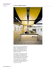### WA1a-12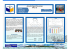### Fiter: Greedy Bloggers for a Picky Audience Avner May, Augustin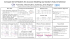### to open - Musicoz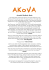### DC Press 2015 copy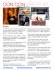### press release - Artnovion Acoustics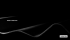### Chitarre Acustiche d`Italia